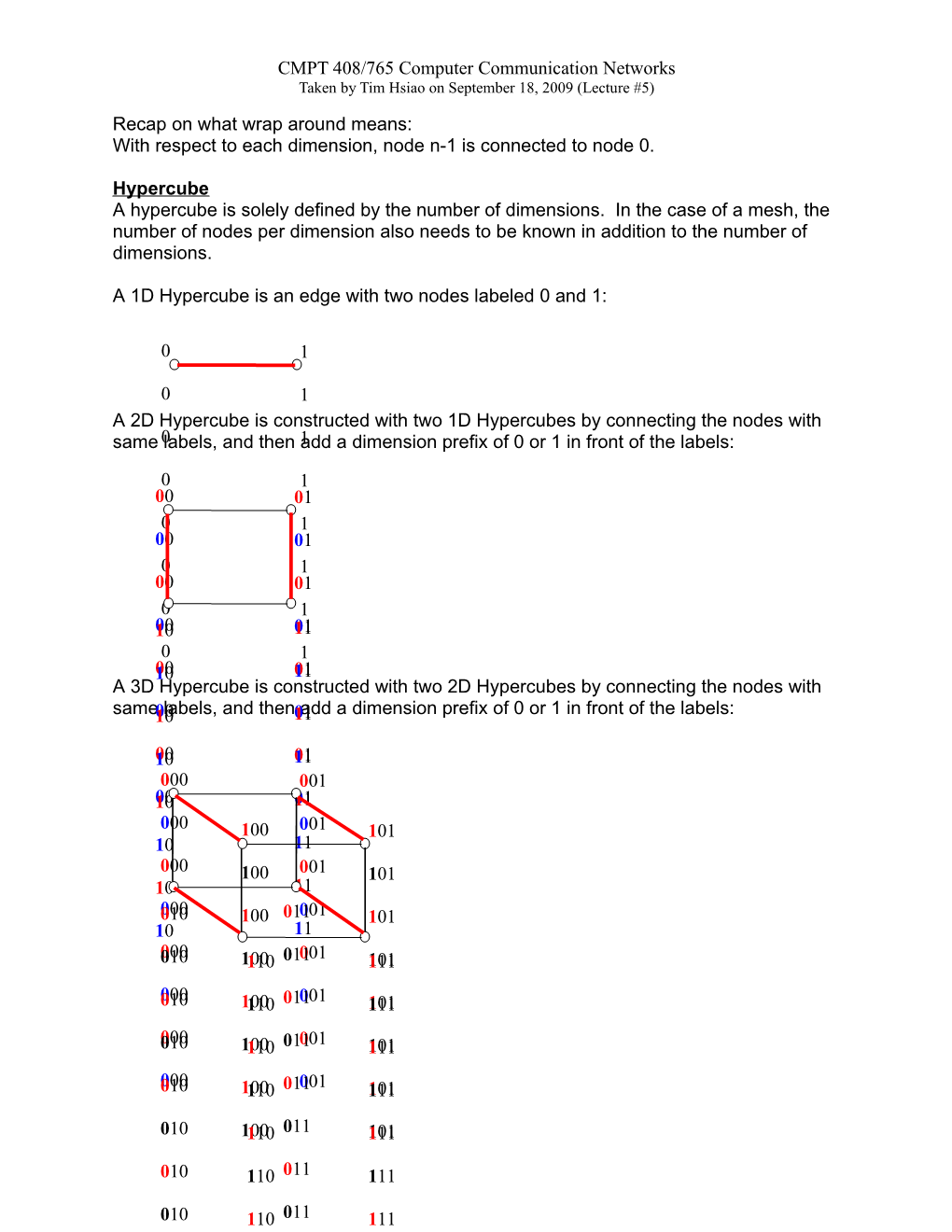# CMPT 408/765 Computer Communication NetworksCMPT 408/765 Computer Communication Networks

Taken by Tim Hsiao on September 18, 2009 (Lecture #5)

Recap on what wrap around means:

With respect to each dimension, node n-1 is connected to node 0.

Hypercube

A hypercube is solely defined by the number of dimensions. In the case of a mesh, the number of nodes per dimensionalso needs to be known in addition to the number of dimensions.

A 1D Hypercube is an edge with two nodes labeled 0 and 1:

A 2D Hypercube is constructed with two 1D Hypercubes by connecting the nodes with same labels, and then add a dimension prefix of 0 or 1 in front of the labels:

A 3D Hypercube is constructed with two 2D Hypercubes by connecting the nodes with same labels, and then add a dimension prefix of 0 or 1 in front of the labels:

A 4D Hypercube is constructed with two 3D Hypercubes:In general, to construct a k-D hypercube, create two k-1D hypercubes, add edges to pairs of nodes with identical labels, and add a prefix of 0 in front of the first node in the pair, and add a prefix of 1 in front of the second node in the pair.

Property of a Hypercube:

Let the G = (V, E) be the graph representation of a k-dimensional hypercube, then an edge (u,v) є E exists if and only if u and v differ by one bit. This implies the Hamming distance, h(u,v) = 1. The Hamming distance between two equal length strings, is the number of positions at which the symbols differ.

Proof by induction:

Basis step-Let k = 1, nodes are labeled 0 and 1, and they differ by one bit.

Inductive Step – For k+1 dimension, we create two k dimensional hypercubes and join nodes of the same label, and then give the nodes a prefix of 0 and 1. Therefore two nodes with edges differ by only one bit.

Let k be the dimension of a hypercube.

Let N be the number of nodes. N = 2k

Let d be the degree of a node. d = k= log2 N

When the dimension of a hypercube is incremented by one, N becomes 2N.

Number of Edges = (N log2 N)/2

Let D be the diameter. D = log2 N = d

d(u,v) = h(u,v) ≤ d

The distance between any two node is d (u,v), in a hypercube it is exactly the Hamming distance h(u,v) between the node labels. It is at most the diameter of the hypercube.

For example, to move from 00110 to 01101, we fix the bits one bit at a time starting from the left most bit. This takes 3 moves. The two labels can differ by at most log2 N bits, therefore diameter is at most log2 N.

A Hamiltonian path in a graph is a path that visits every vertex exactly once. A Hypercube contains a Hamiltonian Path. There are approaches to the proof:

Proof by induction:

Basis (k=1): a one dimensional hypercube contains a Hamiltonian path.

Inductive step: Each of two hypercubes of dimensionk contains a Hamiltonian Path. The two k-dimension hypercubes are joined to form one k+1 dimension hypercube. The path can be joined to form a Hamiltonian Path in k+1 dimension hypercube.Because u and u’ differ by exactly the first bit, there exists an edge between u and u’ in the k+1 dimensional hypercube P. Therefore the path from v to u to u’ to v’ forms a Hamiltonian path in P. Notice there is an edge between v and v’, so a hypercube also contains a Hamiltonian cycle.

Proof by GrayCode (two consecutive binary codes that differ by one bit):

First we generate the following sequence of codes:

0

1

Append the codes in reverse order:

0

1

1

0

For the first half of codes, pad the left most bit with 0. Pad the second half with 1:

00

01

11

10

Perform these two steps recursively to get:

00

01

11

10

10

11

01

00

000

001

011

010

110

111

101

100

Notice that number i differs in 1 bit from i+1 mod number of elements. (Last number differs from first number by one bit as well)

Gray code gives an equivalence of a Hamiltonian Cycle (which decomposes to a path) on a hypercube.

Valiant Routing

Permutation Routing:

V = {v1,v2,v3..., vn}

π V = {vπ1, v π2..., vπn}

• vi will send a message to vπi(for example: 1 2 3 4 5... Nsends to 9 7 3 N 4 ... 6 respectively).
• Each node will send to and receive exactly one packet from one node.
• A node may send a packet to itself.
• Transmission is synchronized; each transmission on a link takes 1 second.
• Each link is capacitated to 1 message each second.
• Multiple message queued at a node is forwarded on a FIFO basis.
• Routing scheme is oblivious (path does not change adaptively).
• Use Left-to-Right bit fixing routing. For instance, to move from 011001 to 010110, the route would be 011001, 010001, 010101, 010111, and 010110.

Problem is that the time spent waiting can greatly exceed maximum path length.